# Down-shifting and filtering a signal

Because the FPGA is fast, but not *that* fast

## Introduction

When I was taking Computer Architecture, I needed an FPGA for the class, and a Basys 3 in particular. In any other year, I would have probably borrowed it from Cal Poly to take computer architecture. However, due to the pandemic, I had to spend money and buy it myself. That thing is \$150 - it ain’t cheap!

Now that class is over, I had this board and I wanted to do something with it. I didn’t want to do something that any ordinary microcontroller could do because that’s boring, in that case I would just use a microcontroller. No, I wanted to make something that requires a FPGA.

So, I thought of making a direct sampling software-defined radio. It won’t have a transmit function, only a receive function, because I have no idea how to do power electronics. Its main purpose would be basically as an ultra wide band scanner (I’m thinking of trying to make it scan a 50 MHz section all at once).

## Problem parameters

The FPGA has a 100 MHz internal clock for most computations, and I want to scan the spectrum of frequencies between 125 and 175 MHz. Therefore, by the Nyquist-Shannon theorem, I will need at least twice that - 325 MSPS (million samples per second) coming in.

I’ve figured out a possible way to get 400 MSPS at an 8 bit resolution into the FPGA. This involves going above 100 MHz, and this will likely be elaborated on in the future. But for now, we’ll just assume for now that that’s the data stream coming in. So, every 100 MHz clock, we get 4 x 8-bit samples. We are dealing with a theoretical data rate of 3.2 Gbps, and this is why we need an FPGA.

## Generating a “raw signal”

Let’s make a signal with lots of different frequency components that we can use for experimenting with. It should reveal why we need to pre-process the signal before FFT.

import numpy as np
import matplotlib.pyplot as plt

# Configuration so that y'all actually see this stuff
plt.rcParams['figure.figsize'] = [11, 7]

M_SAMPLES_PER_SECOND = 400
SAMPLES_PER_SECOND = M_SAMPLES_PER_SECOND * 1e6
NYQUIST = SAMPLES_PER_SECOND / 2
SIGNAL_LENGTH = 10e-3
FFT_SIZE = 4096

# Time domain (1 index = 1 sample at 400 MSPS)
time = np.linspace(0, SIGNAL_LENGTH, SIGNAL_LENGTH * SAMPLES_PER_SECOND)

def tone(x):
return np.cos(x * time * 2 * np.pi)

def fm_tone(x, carrier, deviation):
return np.cos(carrier * time * 2 * np.pi + deviation * np.sin(x * time * 2 * np.pi))

def am_tone(x, carrier):
return (1 + np.cos(x * time * 2 * np.pi)) * np.cos(carrier * time * 2 * np.pi)

received_signal += 10 * am_tone(5e3, 150e6)

# Add in some unwanted tones
received_signal += 0.5 * fm_tone(800, 180e6, 4e3)
received_signal += 4 * fm_tone(250, 50e6, 1e5)

# An increasing frequency tone to act as an indicator
received_signal += 3 * np.cos((time * 100e6 / SIGNAL_LENGTH) * time * 2 * np.pi)

# Add in some noise for the heck of it

bin_size_0 = 1000 * M_SAMPLES_PER_SECOND / 2 / FFT_SIZE

print(f'Bin size: {bin_size_0:02.3f} kHz')

plt.title(f"Full signal ({bin_size_0:02.3f} kHz bins)")
plt.ylabel('frequency (MHz)')
plt.xlabel('time (us)')
plt.show()

Bin size: 48.828 kHz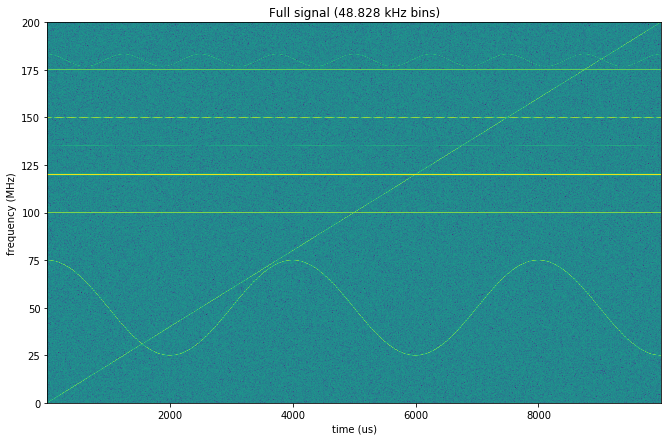Once again, we only care about the 125-175MHz range! We don’t care about anything outside of that! So, all those wonky sine waves I added in are essentially junk data.

Notice that the bin size is 50 kHz. Extremely thick. Narrowband FM on a Baofeng UV-5R is 12.5kHz, and it’s likely even smaller for other radios. We won’t be able to see individual channels, just a group of 4 channels or more.

This is the motivation behind preprocessing the signal. We want to essentially zoom in on the 125-175MHz range so that our FFT can be much more precise for the same amount of computation.

## Shifting the signal downwards

### Bandpass

First, we apply a bandpass filter with cutoffs at 120MHz and 180MHz. They are somewhat offset from the frequencies we want so that we have a little buffer space at the ends.

from scipy import signal

bp_filter = signal.butter(
32,
[120e6 / NYQUIST, 180e6 / NYQUIST],
'band',
analog=False,
output='sos'
)
w, h = signal.sosfreqz(bp_filter, fs=400)
plt.plot(w, 20 * np.log10(abs(h)))
plt.title("Frequency response of the bandpass filter")
plt.ylabel('response (dB)')
plt.xlabel('frequency (MHz)')
plt.show()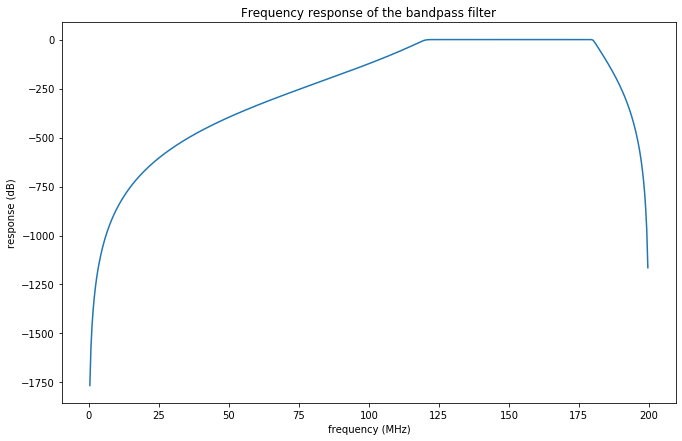bp_sig = signal.sosfilt(bp_filter, received_signal)
plt.specgram(bp_sig, FFT_SIZE, M_SAMPLES_PER_SECOND)
plt.title("Post-Bandpass Signal")
plt.ylabel('frequency (MHz)')
plt.xlabel('time (us)')
plt.show()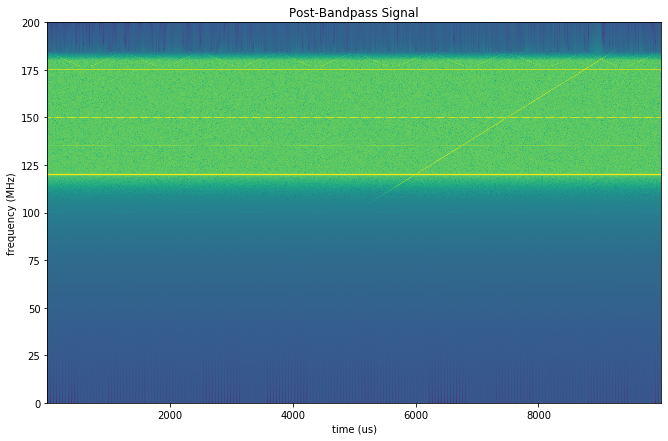### Mixing for Shifting

Next, we mix with a 115MHz signal. For a signal with frequency $f$, this should produce two signals, one at $f - 115\text{MHz}$, and the other reflected at $115\text{MHz} - (f - 115\text{MHz})$.

mixed = bp_sig * np.cos(115e6 * 2 * np.pi * time)

plt.specgram(mixed, FFT_SIZE, M_SAMPLES_PER_SECOND)
plt.title("Post-mixing signal")
plt.ylabel('frequency (MHz)')
plt.xlabel('time (us)')
plt.show()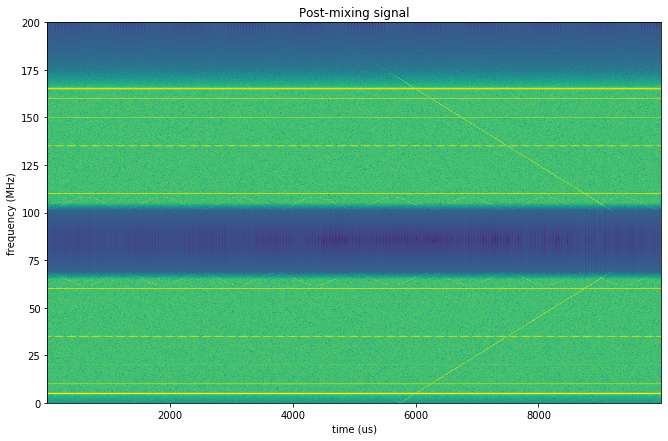### Lowpass

We now perform a low pass to keep the low-frequency signal and get rid of its evil high-frequency twin. The 10-60MHz range here is the equivalent of the original’s 125-175MHz range, so we’ll do a 65MHz lowpass.

lp_filter = signal.butter(
27,
65e6 / NYQUIST,
'lowpass',
output='sos'
)
w, h = signal.sosfreqz(lp_filter, fs=400)
plt.plot(w, 20 * np.log10(abs(h)))
plt.title("Frequency response of the lowpass filter")
plt.ylabel('response (dB)')
plt.xlabel('frequency (MHz)')
plt.show()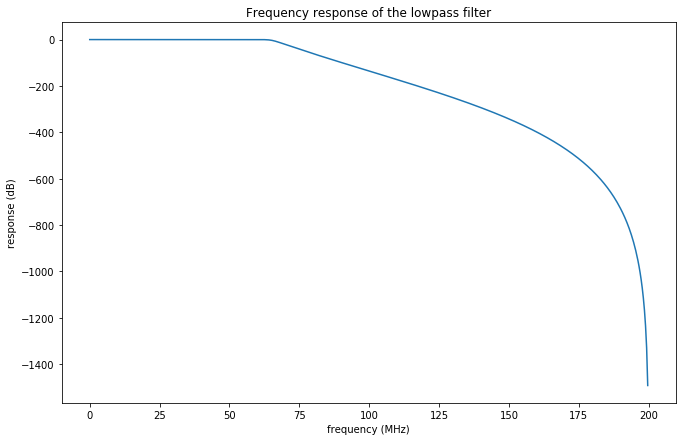lp_sig = signal.sosfilt(lp_filter, mixed)
plt.specgram(lp_sig, FFT_SIZE, M_SAMPLES_PER_SECOND)
plt.title("Post-lowpass signal")
plt.ylabel('frequency (MHz)')
plt.xlabel('time (us)')
plt.show()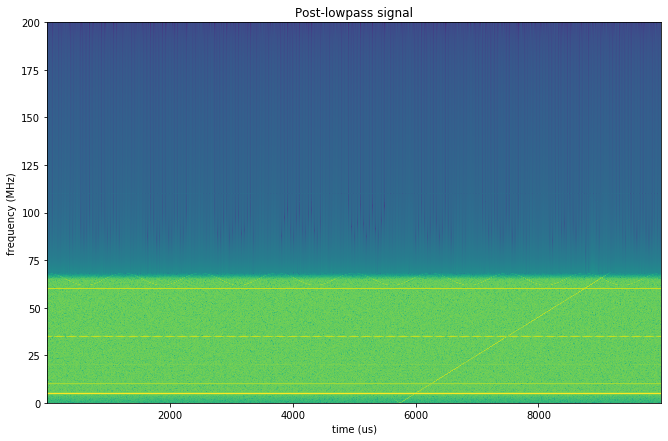### Decimation

Finally, we decimate to get rid of what we don’t want. Decimating by a factor of 3 means that the frequencies included here are between 0-67MHz post-mixing, which is 120-177MHz on the original.

decimate = signal.decimate(lp_sig, 3)
bin_size_1 = 1000 * (M_SAMPLES_PER_SECOND / 3) / 2 / FFT_SIZE

plt.specgram(decimate, FFT_SIZE, M_SAMPLES_PER_SECOND / 3)

plt.title(f"Post-decimate signal ({bin_size_1:02.3f} kHz)")
plt.ylabel('frequency (MHz)')
plt.xlabel('time (us)')
plt.show()

print(f'Bin size (no pre-processing): {bin_size_0} kHz')
print(f'Bin size (with pre-processing): {bin_size_1:02.3f} kHz')Bin size (no pre-processing): 48.828125 kHz
Bin size (with pre-processing): 16.276 kHz

### Cutting off the edges

You can see how the sub-120 MHz signals get aliased in by the “checkmark” over the 1500 tick. This is why we want padding below 125MHz and above 175MHz. However, the aliasing stays below 10 MHz on this spectrogram (125 MHz on the original). So, we’ll just zoom in on the center and pretend the upper and lower bands don’t exist and pretend the axes are the correct “original” values.

plt.specgram(decimate, FFT_SIZE, M_SAMPLES_PER_SECOND / 3)
plt.ylim([10, 60])
plt.title("Final signal that we will use")
plt.ylabel('frequency (MHz)')
plt.xlabel('time (us)')
plt.show()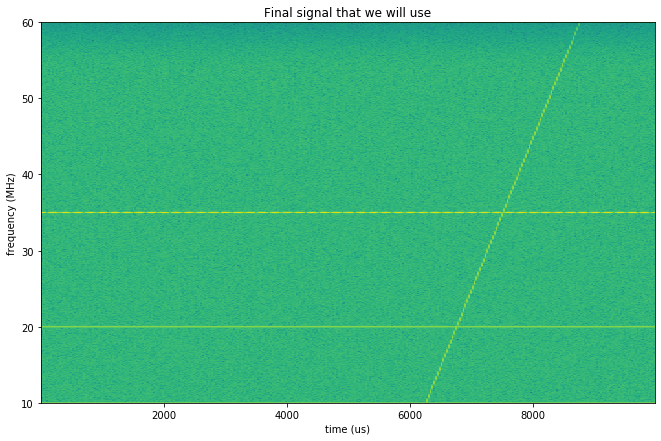## Conclusion

In this post we have designed a method of pre-processing a signal so that performing a FFT on it later will be more precise and have smaller bins. If I continue working on this project, I’ll post updates and make a project page for it.